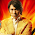Make Questions in Egyptian Dialect

We talked before about how to Make Questions in Standard Arabic. Let's say you want to visit a country like Egypt or have Egyptian friends and want to talk with them in Egyptian dialect. The rule for making questions is a little different in Egyptian dialect. There are different words used and the structure also different.

The structural of questions in Egyptian dialect
While in Standard Arabic, the question word comes in the beginning, in Egyptian dialect, the question word comes in the end. However, sometimes the question word comes in the beginning or in the middle.
Example 1:إنت فِين؟ (= enta fain?: where are you?)
فين (= fain: where) is the question word and it comes in the end. Generally, most the questions in Egyptian dialect are formed with question word in the end.
Example 2:إزاي صحتك؟ (= ezzay sehetak?: How is your health?)
إزاي (= ezzay: how) is the question word and it comes in the beginning.
Example3:عَنْدَك كام سنة؟ (= àandak kam sana?: how old are you?)
كام (= kam: to ask about number) is the question word and it comes in the middle.

The questions words in Egyptian dialect
مين (= meen: who)
فين (= fain: where)
منين (= menain: from where)
على فين (= àala fain: to where)
إيه (= eih: what)
ليه (= laih: why)
إزاي (= ezzay: how)
إمتى (= emta: when)
كام (= kam) used to ask about number. To use it for price, put the preposition بِـ (= be) before the question word to become بِكام (= be kam: how much). See the Infographic below for examples.
أنهي (= anhi: which)
هل (= hal) for yes/no question. Usually, these type of questions come without any question word. Instead, it reveals from the meaning and the way it is said.

Let's test your understanding by this quiz:

Question words in Standard Arabic and in Egyptian dialect:

In Standard Arabic In Egyptian Dialect Translation
مَن (= man) مين (= meen) who
أَيْنَ(= ayna) فين (= fain) where
مِن أَيْنَ (= man ayna) منين (= menain) from where
إلى أَيْنَ(= ela ayna) على فين (= àala fain) to where
مَاذَا (= matha) إيه (= eih) what
لِمَاذَا (= lematha) ليه (= laih) why
كَيْفَ (= kaifa) إزاي (= ezzay) how
مَتَى (= mata) إمتى (= emta) when
أي (= ayy) أنهي (= anhi) which
هل (= hal) هل (= hal) yes/no question

Online Arabic lessons in egyptian dialect
If you need to learn how to speak and understand Egyptian dialect, register in a free trail lesson. You can learn how to speak Egyptian without learning Arabic alphabet.

Sharing is Caring »»

 Tweet

2 التعليقات:

1.2.Ayo Mainkan BvGaming Terbaru dari bolavita... Dengan 1 user ID bisa memainkan semua game yang anda inginkan...

Info Lengkap Hubungi:
WA : 0812-2222-995
Line : cs_bolavita